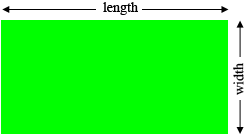SEARCH HOMEMath Central Quandaries & QueriesQuestion from Krunox, a student: A rectangle is twice as long as it is wide. Its perimeter (the sum of the lengths of its sides) is 60 cm. Find its length and width.Hi Krunox,

The challenge here is to set up one or more equation that describes the situation and then solve the equation. My first step is, if possible, to draw a diagram. Here is my diagram.You don't know the length or the width so let's say, let the width of the rectangle be $w$ cm. The length of the rectangle is twice the width so what is the length in terms of $w?$ What is the perimeter in terms of $w?$ But you know the perimeter is 60 cm. Solve for $w.$

PennyMath Central is supported by the University of Regina and the Imperial Oil Foundation.